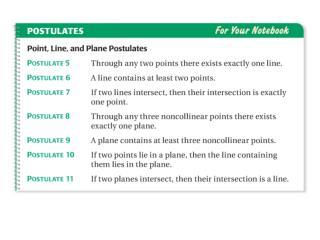Download PresentationEXAMPLE 1# EXAMPLE 1 - PowerPoint PPT Presentation

Download Presentation##### EXAMPLE 1

Download Policy: Content on the Website is provided to you AS IS for your information and personal use and may not be sold / licensed / shared on other websites without getting consent from its author. While downloading, if for some reason you are not able to download a presentation, the publisher may have deleted the file from their server.

- - - - - - - - - - - - - - - - - - - - - - - - - - - E N D - - - - - - - - - - - - - - - - - - - - - - - - - - -
##### Presentation Transcript

1. b. a. a. Postulate 7: If two lines intersect, then their intersection is exactly one point. b. Postulate 11: If two planes intersect, then their intersection is a line. EXAMPLE 1 Identify a postulate illustrated by a diagram State the postulate illustrated by the diagram. SOLUTION

2. EXAMPLE 2 Identify postulates from a diagram Use the diagram to write examples of Postulates 9 and 10. Postulate 9: Plane Pcontains at least three noncollinear points, A, B, and C. Postulate 10: Point Aand point Blie in plane P, so line ncontaining Aand Balso lies in plane P.

3. 1. Use the diagram in Example 2. Which postulate allows you to say that the intersection of plane Pand plane Qis a line? ANSWER Postulate 11 for Example 1 and 2 GUIDED PRACTICE

4. 2. Use the diagram in Example 2 to write examples of Postulates 5, 6, and 7. ANSWER Postulate 5 : Linenpasses through pointsAandB LinencontainsAandB Postulate 6 : Postulate 7 : Linemand n intersect at pointA for Example 1 and 2 GUIDED PRACTICE

5. Sketch a diagram showing TVintersecting PQat point W, so that TWWV. Draw TVand label points Tand V. Draw point Wat the midpoint of TVMark the congruent segments. Draw PQthrough W. EXAMPLE 3 Use given information to sketch a diagram SOLUTION STEP 1 STEP 2 STEP 3

6. Which of the following statements cannot be assumed from the diagram? ABplane S CDplane T AFintersects BCat point B. No drawn line connects E, B, and D, so you cannot assume they are collinear. With no right angle marked, you cannot assume CDplane T. EXAMPLE 4 Interpret a diagram in three dimensions A, B, and Fare collinear. E, B, and Dare collinear. SOLUTION

7. 3. If the given information stated PWand QWare congruent, how would you indicate that in the diagram? ANSWER for Examples 3 and 4 GUIDED PRACTICE In Questions 3 and 4, refer back to Example 3.

8. 4. Name a pair of supplementary angles in the diagram. Explain. ANSWER In the diagram the angle TWP and VWP form a linear pair So, angle TWP, VWP are a pair of supplementary angles. for Examples 3 and 4 GUIDED PRACTICE

9. 5. In the diagram for Example 4, can you assume plane Sintersects plane Tat BC? ANSWER Yes for Examples 3 and 4 GUIDED PRACTICE

10. 6. Explain how you know that ABBCin Example 4. ANSWER It is given that line AB is perpendicular to plane S, therefore line AB is perpendicular to every line in the plane that intersect it at point B. So, AB BC for Examples 3 and 4 GUIDED PRACTICE

11. 1. Use the diagram to write an example of Postulate 7. ANSWER Line m intersects line n in point F and no other point. Daily Homework Quiz For use after Lesson 2.4

12. ANSWER False; Postulate 11 says that if two planes intersect, their intersection is a line. Daily Homework Quiz For use after Lesson 2.4 2. Decide whether the statement “Two planes can intersect in exactly one point R” is true or false. If false, give a reason.

13. Use the diagram to determine if the statement is true or false. 3. The intersection of planes M and N is points B, E and H. ANSWER false Daily Homework Quiz For use after Lesson 2.4

14. 4. GH lies in plane M. ANSWER true Daily Homework Quiz For use after Lesson 2.4 Use the diagram to determine if the statement is true or false.

15. ANSWER false Daily Homework Quiz For use after Lesson 2.4 Use the diagram to determine if the statement is true or false. 5. ABE and HED are vertical angles.